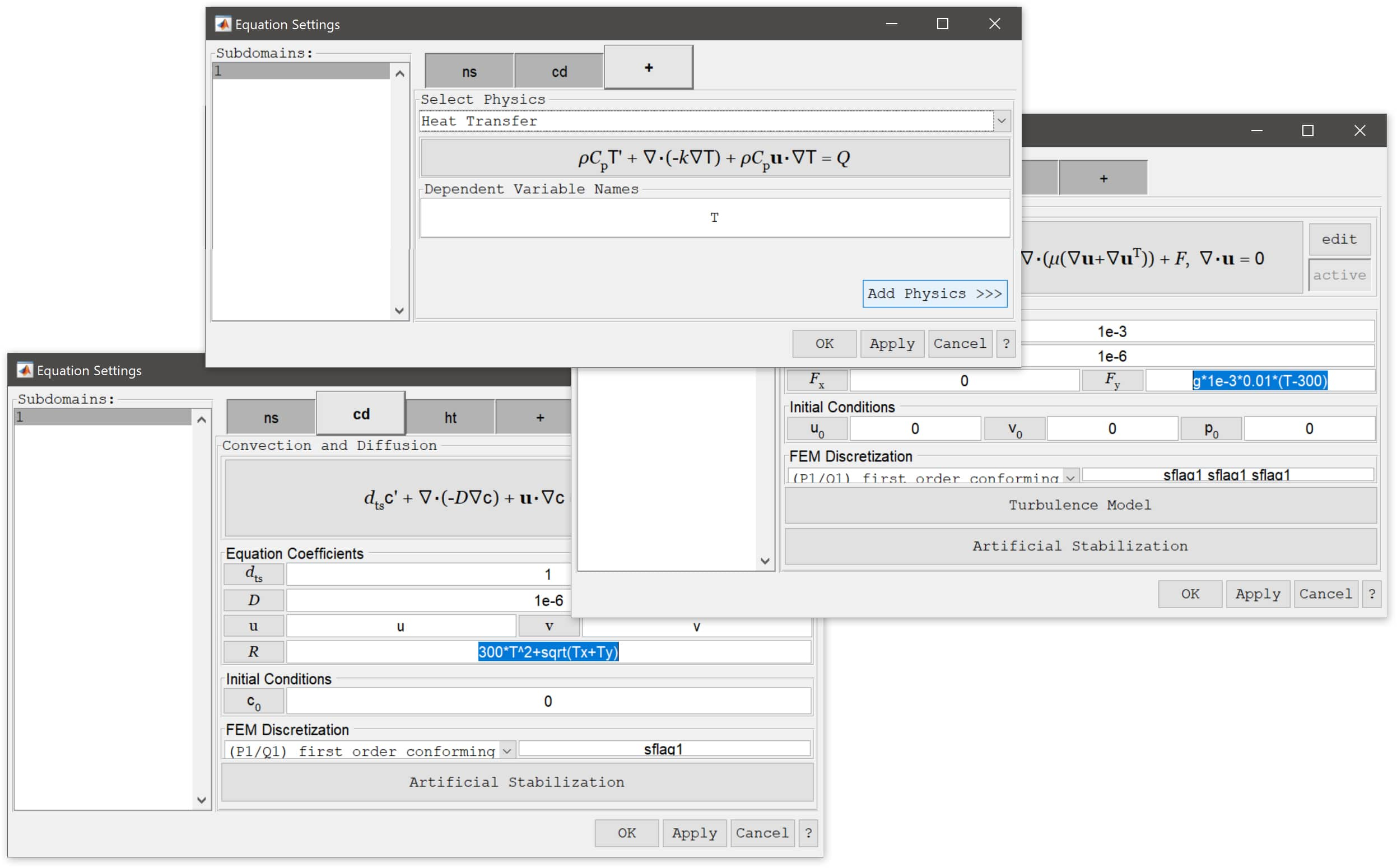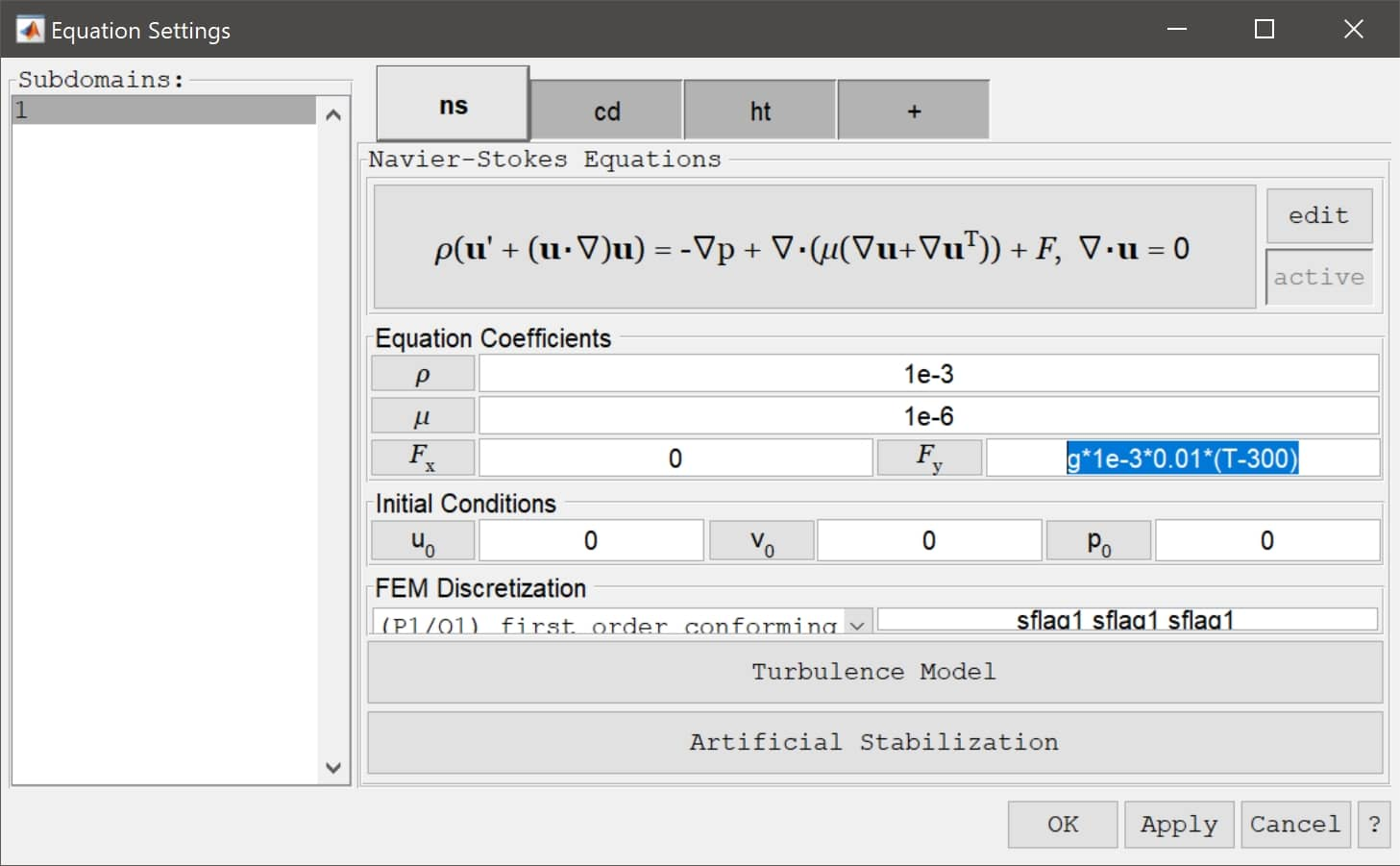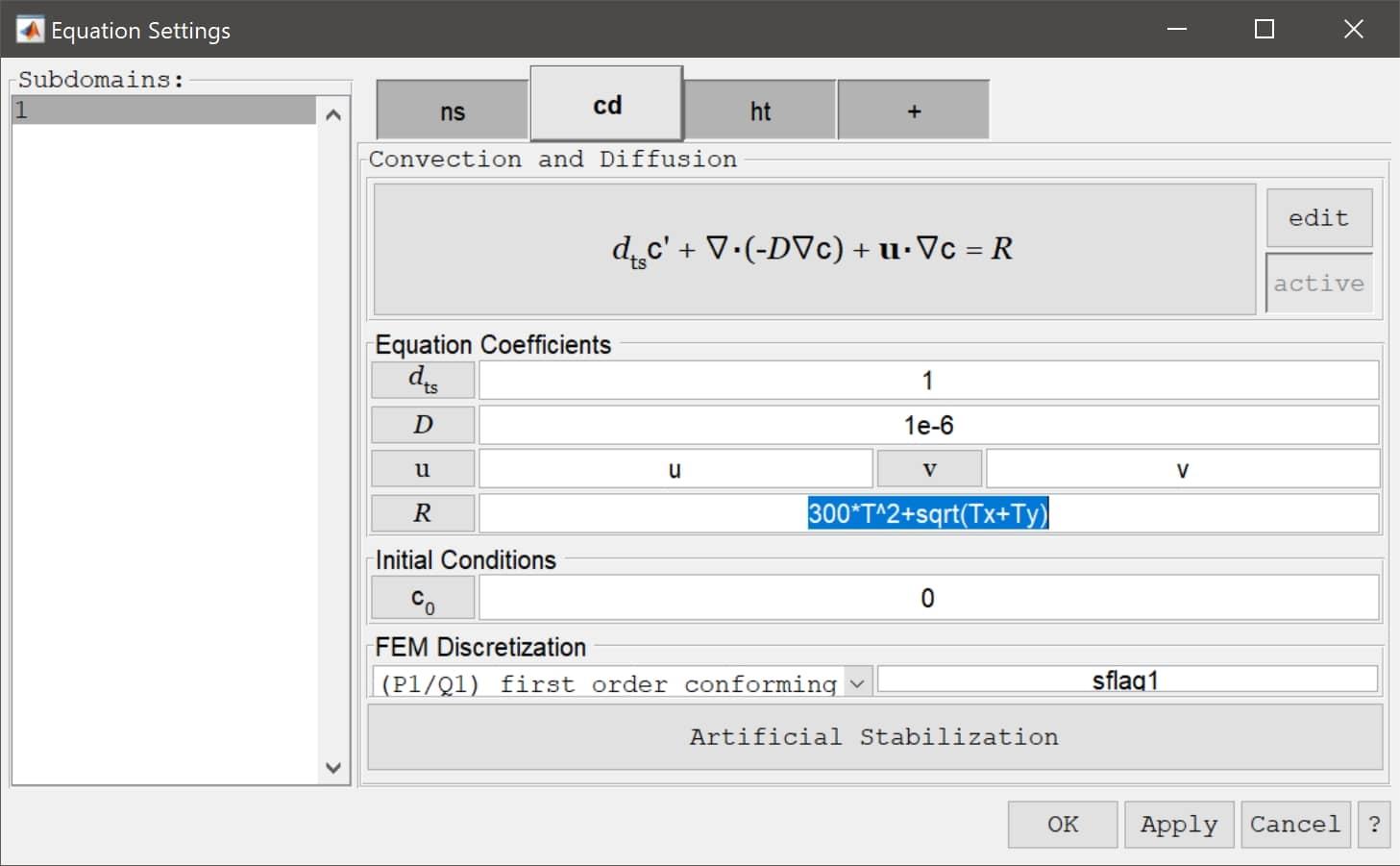# Adding Multiphysics Equations and Using Complex ExpressionsThe FEATool GUI makes it easy to add and coupled multiphysics equations and complex expressions to your models. This post illustrates how to do this.After starting to work with a physics mode it is easy to add one or more other modes by going to the + tab in the Equation Settings dialog box. There you can simply choose an additional physics mode from the drop down combo box, select the dependent variable names you want to use, and press Add Physics >>> to add the additional mode. The new physics mode will now show up as a new tab with its short abbreviated name on its tab. Once the new mode has been added you can switch between the modes by clicking on the corresponding tabs in the Equation and Boundary Settings dialog boxes.

The physics modes can be coupled by simply using the the dependent variable names and derivatives in the coefficient expression dialog boxes. For example, the Navier-Stokes equations physics mode shown below uses the temperature variable T from the heat transfer mode in the source term for the y-direction (F_y).Moreover, the next step illustrates how to make a three way multiphysics coupling. The convective velocities u and v are coupled from the Navier-Stokes equations physics mode and at the same time the temperature T and its two derivatives Tx and Ty are simultaneously coupled to the reaction rate source term in the convection and diffusion mode (R).As we have seen, it is simple to set up multiphysics models in FEATool Multiphysics. This is made possible by the expression parsing functionality that allows entering and using complex expressions of dependent variables (for example u, v, T, c), their first derivatives (by just appending x or y to the variable names like Tx and Ty), the space dimensions x and y, as well as all common MATLAB expressions and constants like pi, sin, cos, log, sqrt, ^2 etc.

Lastly, it is also possible to call user defined m-script functions. If one for example has defined a m-file function called my_function.m which takes two input arguments, then one can simply enter `my_function(x,y)` as an equation coefficient expression which in this case will call the function script with the evaluated values for x and y.

Category: multiphysics